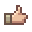# tAPINeed help coding a Beach Ball like item.

#### KingKirmit

##### Golem
So the title says it all, I need to know how to code an item to act like the Vanilla Beach Ball item.
So far the only error is the item not throwing...It uses it but doesn't create the actual projectile.

Any help will be greatly appreciated!#### Sin Costan

##### Eye of Cthulhu
This is from the Vanilla code.

Code:
``````this.timeLeft = 10;
this.ai += 1f;
if (this.ai >= 20f)
{
this.ai = 15f;
for (int num468 = 0; num468 < 255; num468++)
{
Rectangle rectangle3 = new Rectangle((int)this.position.X, (int)this.position.Y, this.width, this.height);
if (Main.player[num468].active)
{
Rectangle value5 = new Rectangle((int)Main.player[num468].position.X, (int)Main.player[num468].position.Y, Main.player[num468].width, Main.player[num468].height);
if (rectangle3.Intersects(value5))
{
this.ai = 0f;
this.velocity.Y = -4.5f;
if (this.velocity.X > 2f)
{
this.velocity.X = 2f;
}
if (this.velocity.X < -2f)
{
this.velocity.X = -2f;
}
this.velocity.X = (this.velocity.X + (float)Main.player[num468].direction * 1.75f) / 2f;
this.velocity.X = this.velocity.X + Main.player[num468].velocity.X * 3f;
this.velocity.Y = this.velocity.Y + Main.player[num468].velocity.Y;
if (this.velocity.X > 6f)
{
this.velocity.X = 6f;
}
if (this.velocity.X < -6f)
{
this.velocity.X = -6f;
}
this.netUpdate = true;
this.ai += 1f;
}
}
}
}
if (this.velocity.X == 0f && this.velocity.Y == 0f)
{
this.Kill();
}
this.rotation += 0.02f * this.velocity.X;
if (this.velocity.Y == 0f)
{
this.velocity.X = this.velocity.X * 0.98f;
}
else
{
if (this.wet)
{
this.velocity.X = this.velocity.X * 0.99f;
}
else
{
this.velocity.X = this.velocity.X * 0.995f;
}
}
if ((double)this.velocity.X > -0.03 && (double)this.velocity.X < 0.03)
{
this.velocity.X = 0f;
}
if (this.wet)
{
this.ai = 0f;
if (this.velocity.Y > 0f)
{
this.velocity.Y = this.velocity.Y * 0.95f;
}
this.velocity.Y = this.velocity.Y - 0.1f;
if (this.velocity.Y < -4f)
{
this.velocity.Y = -4f;
}
if (this.velocity.X == 0f)
{
this.Kill();
}
}
else
{
this.velocity.Y = this.velocity.Y + 0.1f;
}
if (this.velocity.Y > 10f)
{
this.velocity.Y = 10f;
}
}``````
Just got to replace "this" with "projectile". Also, make sure that the item is shooting your projectile.

#### KingKirmit

##### Golem
Is this for the item, or projectile code?
Also is it a json or cs?

#### Sin Costan

##### Eye of Cthulhu
Is this for the item, or projectile code?
Also is it a json or cs?
CS code for Projectile.

#### KingKirmit

##### Golem
Still fails to throw my projectile...
here are the files I have.

The json for the Item
Code:
``````{
"displayName": "Football",
"size": [22,14],
"maxStack": 99,
"value": [0,0,10,0],
"rare": 1,
"tooltip": "Go long! Go long!",
"useStyle": 1,
"useAnimation": 12,
"useTime": 12,
"damage": 0,
"knockBack": 0,
"useSound": 1,
"ranged": true,
"consumable": true,
"shoot": "SwordsofGods:FootballProj",
"shootSpeed": 10,
"noMelee": true
}``````
The json for the Projectile
Code:
``````{
"code": "Football",
"displayName": "Football",
"size": [22,14],
"aiStyle": 155,
"timeLeft": 10,
"friendly": true,
"hostile": false,
"tileCollide": true,
"damage": 0,
"penetrate": 0,
"ranged": true
}``````
The cs for the Projectile
Code:
``````using System;
using Microsoft.Xna.Framework;
using Microsoft.Xna.Framework.Graphics;

using TAPI;
using Terraria;

namespace SwordsofGods

{

public class Football : ModProjectile
{

public override void PostAI()
{
projectile.timeLeft = 10;
}
}
}``````

#### Sin Costan

##### Eye of Cthulhu
Still fails to throw my projectile...
here are the files I have.

The json for the Item
Code:
``````{
"displayName": "Football",
"size": [22,14],
"maxStack": 99,
"value": [0,0,10,0],
"rare": 1,
"tooltip": "Go long! Go long!",
"useStyle": 1,
"useAnimation": 12,
"useTime": 12,
"damage": 0,
"knockBack": 0,
"useSound": 1,
"ranged": true,
"consumable": true,
"shoot": "SwordsofGods:FootballProj",
"shootSpeed": 10,
"noMelee": true
}``````
The json for the Projectile
Code:
``````{
"code": "Football",
"displayName": "Football",
"size": [22,14],
"aiStyle": 155,
"timeLeft": 10,
"friendly": true,
"hostile": false,
"tileCollide": true,
"damage": 0,
"penetrate": 0,
"ranged": true
}``````
The cs for the Projectile
Code:
``````using System;
using Microsoft.Xna.Framework;
using Microsoft.Xna.Framework.Graphics;

using TAPI;
using Terraria;

namespace SwordsofGods

{

public class Football : ModProjectile
{

public override void PostAI()
{
projectile.timeLeft = 10;
}
}
}``````
You forgot the rest of the cs code.I forgot to remove all that spacing, so you might have to do that. Make sure you copy the whole code.

•G4M3R57

#### KingKirmit

##### Golem
Oppsmissed all the other codding...
but still fails to throw my projectile...I triple even quad checked the names of the files and made sure everything matched...

•G4M3R57

#### Wulf'frid

##### Terrarian
Oppsmissed all the other codding...
but still fails to throw my projectile...I triple even quad checked the names of the files and made sure everything matched...
as I recall, projectile.timeleft works in ticks, so 10, isn't very long at all

•G4M3R57